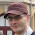## Sunday, May 30, 2010

### Sub-array indexing

One of the things I have been missing in CL was convenient indexing for sub-arrays. Languages like R and Octave all have convenient sub-array indexing. I have experimented with various designs before (some of them I made public, eg AFFI and xarray), but none of them were entirely satisfactory and I kept searching for new solutions.

This is primarily an issue of syntactic convenience: I want to be able to select elements from arrays without writing (nested) loops. I also want to be able to copy elements from arrays to other arrays, similarly selected. Speed is welcome, but at this stage it is secondary: so far, profiling indicates that these operations comprise a tiny fraction of execution time in my programs, but having the ability to capture operations using (sub)arrays is an enormous timesaver and an invaluable semantic abstraction.

## Introduction to sub

My latest attempt at tackling this problem is the generic function sub in my cl-num-utils library (BTW, this library is the successor of my earlier cl-numlib, which is now deprecated, all useful functionality will end up in other libraries, eventually). The syntax is as follows: (sub object &rest ranges). Each range is either a fixnum (selecting a single index), (cons start end) (selecting indexes in that range, excluding end as is usually done for CL library functions), t for all indexes, and finally, a vector of fixnums for anything else.

Let's see some examples:

CLNU> (defparameter *a* #2A((1 2 3 4) (5 6 7 8) (9 10 11 12)))
*A*
CLNU> *a*
#2A((1 2 3 4) (5 6 7 8) (9 10 11 12))
CLNU> (sub *a* 1 t) ; second row
#(5 6 7 8)
CLNU> (sub *a* '(1 . 2) t) ; second row as matrix
#2A((5 6 7 8))


Notice how a single fixnum will drop that dimension (making a vector from a matrix), while selecting the same row with a cons doesn't.

For all other cases, you can use vectors:

CLNU> (sub *a* t #(0 3)) ; first and last columns
#2A((1 4) (5 8) (9 12))
CLNU> (sub *a* t #(3 0)) ; last and first columns
#2A((4 1) (8 5) (12 9))


You can also use negative numbers for indexes: if (minusp index), it will select the column (- dimensions index):

CLNU> (sub *a* -1 t) ; last row
#(9 10 11 12)
CLNU> (sub *a* #(-2 -1) #(-2 -1)) ; bottom right 2x2 matrix
#2A((7 8) (11 12))


Finally, 0 in a cons corresponds to the largest possible index in that dimension:

CLNU> (sub *a* '(-2 . 0) t) ; last two rows
#2A((5 6 7 8) (9 10 11 12))


## Setting subarrays

sub can also be used with setf:

CLNU> *a*
#2A((1 2 3 4) (5 6 7 8) (9 10 11 12))
CLNU> (setf (sub *a* 1 t) #(-1 -2 -3 -4)) ; replace second row
#(-1 -2 -3 -4)
CLNU> *a*
#2A((1 2 3 4) (-1 -2 -3 -4) (9 10 11 12))
CLNU> (setf (sub *a* 2 t) (map 'vector #'+ (sub *a* 0 t) (sub *a* 1 t)))
#(0 0 0 0)
CLNU> *a*
#2A((1 2 3 4) (-1 -2 -3 -4) (0 0 0 0))


Note that the ranks have to be equal, make sure that you keep/drop dimensions as needed.

## Macros for implementing sub

In cl-num-utils, you also find the macro with-range-indexing, which preprocesses the range arguments and implements an internal counter for row-major indexing. For example, sub for arrays is implemented as

(defmethod sub ((array array) &rest ranges)
(declare (optimize debug (speed 0)))
(with-range-indexing (ranges (array-dimensions array) next-index
:end? end?
:range-dimensions dimensions)
(let ((result (make-array (coerce dimensions 'list)
:element-type
(array-element-type array))))
(iter
(until end?)
(for result-index :from 0)
(setf (row-major-aref result result-index)
(row-major-aref array (next-index))))
result)))


The macro takes the range specification as the ranges argument, and also needs the original dimensions of the array. next-index should be the name of the function that will be used to query the next index: it increments the internal counter and delivers the next index. The internal counter is an array of fixnums, and the index is calculated with an affine mapping, but the algorithm allows to update the sum only for the indexes which actually changed. All this is hidden by the macro. end? is a boolean, which can be used to query when all the elements have been traversed. dimensions will be bound to the dimensions of the result, after allowing for dimension droppings.

This macro is pretty versatile: I used it in LLA to implement (sub dense-matrix-like ...), ((setf sub) array dense-matrix-like ...) and ((setf sub) dense-matrix-like array ...) methods, even though LLA is column-major. The trick is to swap dimensions.

1.I just installed cl-num-utils (cl-num-utils-20131211-git) via quicklisp but could not find the sub function. Was it renamed or moved to another package?

1.See https://github.com/tpapp/cl-slice. This appears to be a later T Papp library with sub-array slicing capabilities.

2.See https://github.com/tpapp/cl-slice